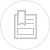# general points on semi-open type cooling circuits

The characteristic elements of a semi-open circuit are as follows:

• the circuit volume: V (m3);
• cooling water flow: Q (m3/h);
• the variation in temperature of water to be cooled between the tower inlet and outlet:

DT (°C) = outlet temperature – inlet temperature;

• the power of the tower : W (kcal/h) = Q x Dt x 1,000;
• evaporation (quantity of water evaporated from the tower): E (m3/h)

To transform 1 kilogram of water into steam, 560 kcal are required (latent heat of vaporisation).

Calories will mostly be eliminated by the change in state of the water from the liquid phase to the vapour phase.

This results in the calculation of evaporation via the formula (considering that 100% of the exchange occurs via the recovery of the latent heat of vaporisation):

The water transformed into vapour is pure and does not contain any mineral salts.

#### drift droplets (water droplets entrained in the atmosphere) : Ev (m3/h)

This water contains mineral salts. It has the same chemical composition as water from the circuit and as a consequence, must be taken into account in the blowdown calculation. In general, we accept that:

#### the concentration ratio (also known as concentration factor): K

It is the ratio of the flow of make-up water and the flow of blowdown water which must correspond to the ratio of dissolved salt content in the make-up water.

The ability to concentrate the water means water consumption and discharge are reduced. As indicated in the graph below, concentration will depend on the mineral content of the water used.

#### blowdown water: P (m3/h)

Water evaporation from the tower results in an increased concentration of salts in the circulating water. This increase must be limited if mineral precipitation is to be avoided. It is therefore necessary to discharge a part of the water in circulation into a drain (de-concentration). The blowdown water flow can be calculated using the formula:

#### make-up water: A (m3/h)

It complements the volume of water in the circuit to be maintained at a constant level by compensating losses resulting from evaporation, drift and purging. Make-up water can be calculated using the formula:

#### maximum tube skin temperature

It is the temperature of the water film in contact with the hottest wall in the circuit.

#### half-residence time: T1/2

This equates to the time needed for the concentration of an injected solute to be reduced by half. The half-residence time is calculated using the formula:

Based on the physicochemical characteristics of the make-up water, it is possible to calculate the Ryznar Index ( RI ) in the circuit according to a given temperature. This index allows the assessment of water behaviour, whether it is scaling (precipitation of mineral salts in excess) or aggressive (capacity to dissolve minerals). Based on the measured pH of the water, pHo, and the saturation pH , pHs, we can calculate the Ryznar Index for a given temperature.

The Ryznar Index is defined in the following manner: